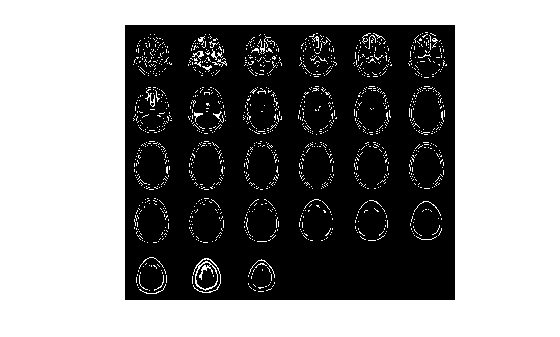# edge3

Find edges in 3-D grayscale volume

## Syntax

``BW = edge3(V,"approxcanny",thresh)``
``BW = edge3(V,"approxcanny",thresh,sigma)``
``BW = edge3(V,"Sobel",thresh)``
``BW = edge3(V,"Sobel",thresh,"nothinning")``

## Description

````BW = edge3(V,"approxcanny",thresh)` returns the edges found in the grayscale or binary volume `V` using the approximate Canny method. The approximate Canny method finds edges by looking for local maxima of the gradient of `V`. `edge3` calculates the gradient using the derivative of a Gaussian smoothed volume.The approximate Canny method uses two thresholds to detect strong and weak edges, and includes the weak edges in the output only if they are connected to strong edges. This method is more likely than the Sobel method to detect true weak edges.```

example

````BW = edge3(V,"approxcanny",thresh,sigma)` returns the edges found in the intensity or binary volume `V`, where `sigma` specifies the standard deviation of the Gaussian smoothing filter. `edge3` chooses the size of the filter automatically, based on `sigma`.```
````BW = edge3(V,"Sobel",thresh)` accepts an intensity or a binary volume `V` and returns a binary volume `BW` with `1`s where the function finds edges in `V` and `0`s elsewhere.The Sobel method finds edges using the Sobel approximation to the derivative. It returns edges at those points where the gradient of `V` is maximum. `edge3` ignores all edges that are not stronger than `thresh`.```
````BW = edge3(V,"Sobel",thresh,"nothinning")` speeds up the operation of the algorithm by skipping the additional edge-thinning stage. By default, or when `"thinning"` is specified, `edge3` applies edge thinning.```

## Examples

collapse all

Load volumetric data and remove any singleton dimensions.

```load mri V = squeeze(D);```

Visualize original image.

`montage(reshape(V,size(D)),map);`Detect edges in the volume.

`BW = edge3(V,'approxcanny',0.6);`

Visualize the detected edges. You can also view the result using the Volume Viewer app.

`montage(reshape(BW,size(D)))`## Input Arguments

collapse all

Input volume, specified as a 3-D numeric array.

Data Types: `single` | `double` | `int8` | `int16` | `int32` | `int64` | `uint8` | `uint16` | `uint32` | `uint64` | `logical`

Sensitivity threshold, specified as one of the following.

MethodThreshold value
CannyNumeric scalar
Approximate Canny2-element numeric row vector. The first element is the low threshold, and the second element is the high threshold, `[lowthresh highthresh]`
Numeric scalar representing the high threshold. `edge3` sets the low threshold as `0.4*thresh`.
Sobel

Numeric scalar

Data Types: `single` | `double` | `int8` | `int16` | `int32` | `int64` | `uint8` | `uint16` | `uint32` | `uint64` | `logical`

Standard deviation of the Gaussian filter, specified as a numeric scalar for isotropic volumes or a 1-by-3 numeric vector of the form `[SigmaX SigmaY SigmaZ]` for anisotropic volumes that have different scales in each direction.

Data Types: `single` | `double` | `int8` | `int16` | `int32` | `int64` | `uint8` | `uint16` | `uint32` | `uint64` | `logical`

## Output Arguments

collapse all

Detected edges, returned as a 3-D numeric array of the same size as `V`. Pixel values of `1` indicate edges and pixel values of `0` indicate flat regions.

## Version History

Introduced in R2017b

expand all